Next: Heisenberg's Uncertainty Principle Up: Fundamentals of Quantum Mechanics Previous: Operators

Momentum Representation

Fourier's theorerm (see Sect. 3.12), applied to one-dimensional wavefunctions, yields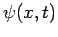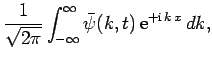(200)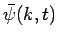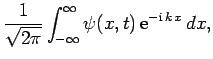(201)

whererepresents wavenumber. However,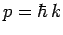. Hence, we can also write(202)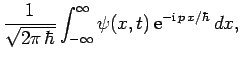(203)

whereis the momentum-space equivalent to the real-space wavefunction.

At this stage, it is convenient to introduce a useful function called the Dirac delta-function. This function, denoted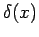, was first devised by Paul Dirac, and has the following rather unusual properties:is zero for, and is infinite at. However, the singularity atis such that(204)

The delta-function is an example of what is known as a generalized function: i.e., its value is not well-defined at all, but its integral is well-defined. Consider the integral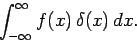(205)

Sinceis only non-zero infinitesimally close to, we can safely replace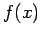by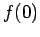in the above integral (assumingis well behaved at), to give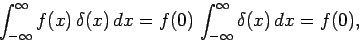(206)

where use has been made of Eq. (204). A simple generalization of this result yields(207)

which can also be thought of as an alternative definition of a delta-function.

Suppose that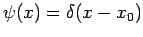. It follows from Eqs. (203) and (207) that(208)

Hence, Eq. (202) yields the important result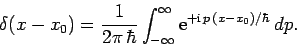(209)

Similarly,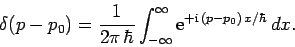(210)

It turns out that we can just as well formulate quantum mechanics using momentum-space wavefunctions,, as real-space wavefunctions,. The former scheme is known as the momentum representation of quantum mechanics. In the momentum representation, wavefunctions are the Fourier transforms of the equivalent real-space wavefunctions, and dynamical variables are represented by different operators. Furthermore, by analogy with Eq. (192), the expectation value of some operatortakes the form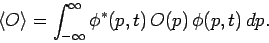(211)

Consider momentum. We can write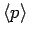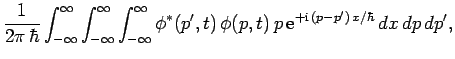(212)

where use has been made of Eq. (202). However, it follows from Eq. (210) that(213)

Hence, using Eq. (207), we obtain(214)

Evidently, momentum is represented by the operatorin the momentum representation. The above expression also strongly suggests [by comparison with Eq. (158)] that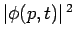can be interpreted as the probability density of a measurement of momentum yielding the valueat time. It follows thatmust satisfy an analogous normalization condition to Eq. (140): i.e.,(215)

Consider displacement. We can write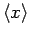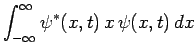(216)Integration by parts yields(217)

Hence, making use of Eqs. (210) and (207), we obtain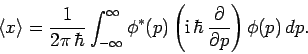(218)

Evidently, displacement is represented by the operator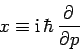(219)

in the momentum representation.

Finally, let us consider the normalization of the momentum-space wavefunction. We have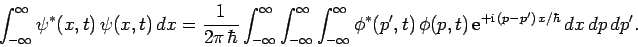(220)

Thus, it follows from Eqs. (207) and (210) that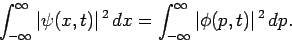(221)

Hence, ifis properly normalized [see Eq. (140)] then, as defined in Eq. (203), is also properly normalized [see Eq. (215)].

The existence of the momentum representation illustrates an important point: i.e., that there are many different, but entirely equivalent, ways of mathematically formulating quantum mechanics. For instance, it is also possible to represent wavefunctions as row and column vectors, and dynamical variables as matrices which act upon these vectors.Next: Heisenberg's Uncertainty Principle Up: Fundamentals of Quantum Mechanics Previous: Operators
Richard Fitzpatrick 2010-07-20